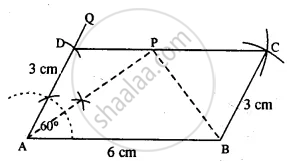# Using Ruler and Compasses Only, Construct a Parallelogram Abcd, in Which : Ab = 6 Cm, Ad = 3 Cm and ∠Dab = 60°. in the Same Figure Draw the Bisector of Angle Dab and Let It Meet Dc at Point P. - Mathematics

Sum

Using ruler and compasses only, construct a parallelogram ABCD, in which : AB = 6 cm, AD = 3 cm and ∠DAB = 60°. In the same figure draw the bisector of angle DAB and let it meet DC at point P. Measure angle APB.

#### SolutionSteps :

1. Draw AB = 6 cm.
2. At A draw ∠QAB = 60°.
3. From AQ cut AD = 3 cm.
4. From D, draw an arc of radius 6 cm.
5. From B, draw an arc of radius 3 cm which meets the first arc at C.
6. Join CD and BC.
Thus ABCD is the required ||gm.
7. Bisect ∠DAB, so that bisector meets CD at P.
8. Join PB and measure ZAPB.
∴ ∠APB = 90°.
Concept: Construction of Parallelograms
Is there an error in this question or solution?

#### APPEARS IN

Selina Concise Mathematics Class 8 ICSE
Chapter 18 Constructions
Exercise 18 (D) | Q 8 | Page 212